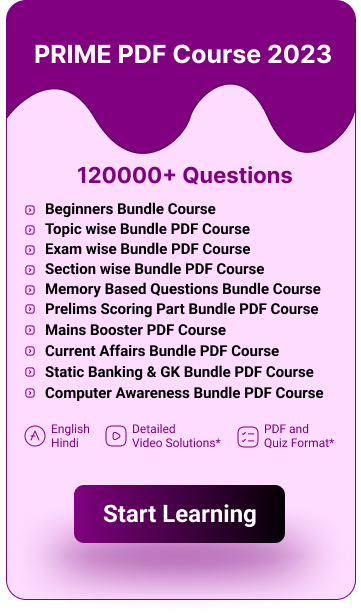# Average Tricks and Shortcuts For Bank PO and Clerk Exams

Average Tricks and Shortcuts For Bank Exams: Average tricks and shortcuts for bank exams is provided here in this average tricks and shortcuts for bank exams  article. Aspirants who are effectively preparing for their upcoming bank exams and other competitive exams like ssc cgl, ssc chsl, ssc mts, railways and more can go through this average tricks and shortcuts for bank exams article. Average is one of the most interesting and important topics in the quantitative aptitude section of the bank exams and other competitive exams. In most of the bank exams like sbi clerk, sbi po, ibps clerk, ibps po, rrb clerk, rrb po and other insurance exams, it is impossible to see the question paper without these average questions. So the aspirants who are going to attend the bank exams must know some average tricks and shortcuts for bank exams. These average tricks and shortcuts for bank exams will help you to make your calculations simpler while solving the average questions by using these average tricks and shortcuts for bank exams. Average tricks and shortcuts for bank exams will also help you to improve your speed and accuracy.

## Average Tricks and Shortcuts For Bank Exams

Here we have added some basic average tricks and shortcuts in this post. Candidates before going to practice various types of average questions they should know some basic average tricks and shortcuts for bank exams. It will improve your speed and accuracy and make your calculations easier while solving the average questions with average tricks and shortcuts for bank exams. To calculate the average questions you can use the average tricks and shortcuts which is given below.

• Average is calculated by dividing the sum of all values by the total number of values.

Average Formula = Sum of all values / Total number of values

• First and foremost the candidates need to find out all values in the average question.
• If no value is given in the question try to find out the total number of elements by using the average formula for bank exams or by downloading the average formula pdf for bank exams.
• Practicing various types of average questions regularly on a daily basis is the key to ace the average questions in the bank exams.
• You need to be knowledgeable with the average formula for bank exams and average tricks and shortcuts for bank exams to make your calculations easier in the bank exams and other competitive exams. You can download the average formula pdf for bank exams here for your reference.

Average formula pdf for bank exams

 More Quantitative Aptitude Formula PDFs Percentage Average Probability Time Distance Speed Time and Work Permutation and Combination Boats and Streams Simple Interest and Compound Interest Number System Mixture and Alligation Ratio and Proportion Pipes and Cisterns Sequence and Series Problems on Trains Partnership Profit and Loss

## Average Tricks and Shortcuts For Bank Exams FAQs

Q. What is the formula to find average?

A. Average Formula = Sum of all values / Total number of values

Q. What are the tricks and shortcuts to find average in bank exams?

A. Kindly go through the above average tricks and shortcuts for bank exams article to know the tricks and shortcuts to find average.

• TAGS

Jun 10 2023

Jun 10 2023

Jun 10 2023

## Don’t Miss Any Update

Enter the valid e-mail id and subscribe to Guidely to get the latest updates on all job notifications, Study Materials, Mock Tests and our new launches.

###### Platinum Package
• 3000+ Mock Tests
• Topic / Sectional / ebooks
• Latest Exam Pattern
• 12 Months
• Access Mobile app and Website